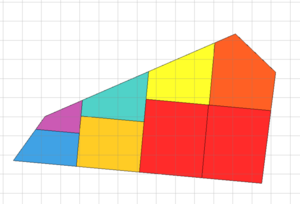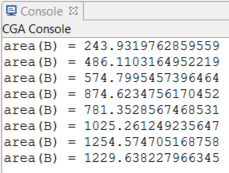# splitArea operation

### Syntax

• splitArea(axis) { area1 : operations1 | area2 : operations2 | ... | arean-1 : operationsn-1 }
• splitArea(axis) { area1 : operations1 | area2 : operations2 | ... | arean-1 : operationsn-1 }*
• splitArea(axis, adjustMode) { area1 : operations1 | ... | arean-1 : operationsn-1 }
• splitArea(axis, adjustMode) { area1 : operations1 | ... | arean-1 : operationsn-1 }*

### Parameters

1. axisselector
{ x | y | z }—Name of axis to split along. This is relative to the local coordinate system (i.e. the scope).
{ adjust | noAdjust }—Optional selector to control scope calculation of the calculated shapes. The default is to adjust the scope to the geometry's bounding box. Using noAdjust avoids this, and therefore, the scopes of the resulting shapes fill the parent's scope without gaps.
3. areafloat
Split area. The current shape is split such that the resulting shape has the specified area. Depending on the prefix, the area is interpreted in the following way:
• no prefix(absolute) —The new shape will have exactly the same area.
• ' (relative) —The new shape's size will be area * current geometry's area.
• ~ (floating) —With the ~ prefix the remaining spaces between the split parts with absolute dimensions are automatically adapted. If multiple floating parts are defined within a split, the dimensions are weighed proportionally.
4. operations
A sequence of shape operations to execute on the newly created shape.
5. *
Repeat switch: the repeat switch triggers the repetition of the defined split into the current shape's scope, as many times as possible. The number of repetitions and floating dimensions are adapted to the best solution (best number of repetitions and least stretching).

### Description

The splitArea operation subdivides the geometry of the current shape along the specified scope axis into a set of shapes with geometric areas specified by the area parameter. This operation works on planar 2D geometry and almost planar geometry. The area accuracy breaks down with nonplanar geometry. This operation does not apply to 3D geometry such as meshes or highly nonplanar geometry.

For each specified area, the current shape's geometry is cut with planes perpendicular to the split axis such that the resulting shape contains geometry with the specified area.

The optional repeat switch * can be used to repeat the content within a { ... }* block as many times as it fully fits into the scope's dimension along the selected axis.

### Examples

#### Colors

These are the rules for the colors used in the following examples.

``````Red     -->                          // red
color(1, 0, 0)
print("area(R) = " + geometry.area)

Yellow  -->                         // yellow
color(1, 1, 0)
print("area(Y) = " + geometry.area)

Green   -->                         // green
color(0, 1, 0)
print("area(G) = " + geometry.area)

Cyan    -->                         // cyan
color(0, 1, 1)
print("area(C) = " + geometry.area)

Blue    -->                         // blue
color(0, 0, 1)
print("area(B) = " + geometry.area)

Magenta -->                         // magenta
color(1, 0, 1)
print("area(M) = " + geometry.area)

Pink    -->                         // semi-transparent pink
color(1, 0.5, 0.5, 0.3)``````
 This shape is split into two parts. The first part is 70% of the original area, and the second part is 30% of the original area. Note that the specified area amounts do not need to sum to 1. For sums less than 1, the rest of the shape is discarded, and split sections greater than 1 are ignored. ``````Lot --> print("total area = " + geometry.area) splitArea(x) { '0.7 : Green | '0.3 : Yellow }``````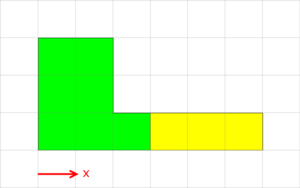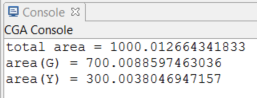#### Repeating Split

 This split divides the shape into five equal area parts using a repeat split. It repeatedly splits the shape into parts with area equal to 20% of the original area. Note that if area were set to '0.3, we would get four parts with areas equal to 30%, 30%, 30%, and 10% of the original area.``````Lot --> print("total area = " + geometry.area) splitArea(x) { '0.2 : ColorMe }* ColorMe --> case split.index == 0 : Red case split.index == 1 : Yellow case split.index == 2 : Green case split.index == 3 : Cyan else : Blue``````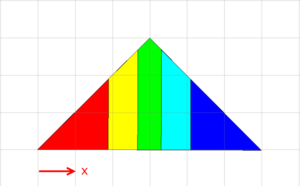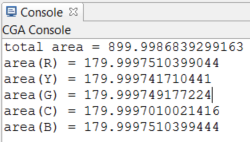#### Split with mixture of floating and absolute areas

 This split divides the shape into a middle part (yellow) that has an absolute area of 600 and two side parts each with the same area. The yellow part consists of two pieces since there is a hole in the original shape, and the split cuts the shape along the hole boundary. ``````Lot --> print("total area = " + geometry.area) splitArea(x) { ~1 : Green | 600 : Yellow | ~1 : Blue``````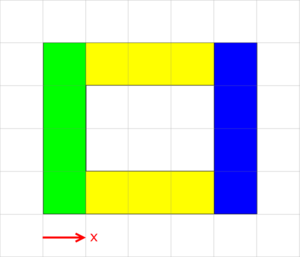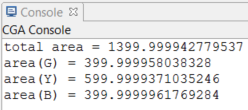#### Nested Split

 This nested split divides the shape first in x to get three equal area parts. Then, each part is divided in z into two equal parts. This yields six equal area parts. ``````Lot --> print("total area = " + geometry.area) splitArea(x) { ~1 : splitArea(z) { '0.5 : Red | '0.5 : Yellow } | ~1 : splitArea(z) { '0.5 : Green | '0.5 : Cyan } | ~1 : splitArea(z) { '0.5 : Blue | '0.5 : Magenta } }``````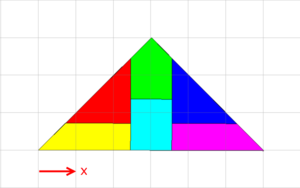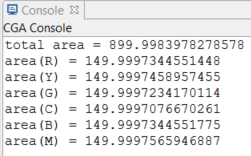This is the original shape and its scope.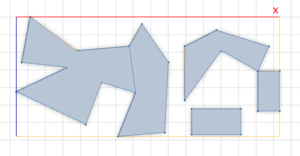Without specifying a value for adjustSelector, the default behavior is to adjust the scope size to the geometry of each split part. Each pink box shows the scope of each split part. The scope maintains the original scope's orientation but shrinks to the bounding box of the geometry. ``````SplitAdjust --> splitArea(x) { '0.2 : splitArea(z) { '0.2 : SplitLeaf }* }* SplitLeaf--> color(0.5, 0.5, 0.5) Geometry. primitiveQuad() t(0, 0.02, 0) Pink comp(e) { all : color(0, 0, 0) Edge. }``````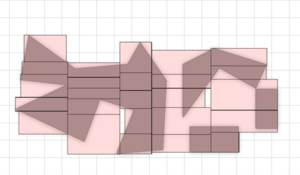With noAdjust, the union of the scopes of each split part make up the original scope. The scopes are not adjusted to the geometry inside. `````` SplitNoAdjust --> splitArea(x, noAdjust) { '0.2 : splitArea(z, noAdjust) { '0.2 : SplitLeaf }* }*``````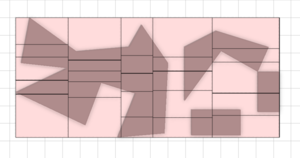This is the original shape and its scope. Here is an example of how the splitArea operation can be used to divide a street block into lots with equal areas.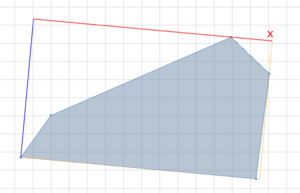The block is divided recursively three times using splitArea. Each split divides the shape perpendicular to its longest axis into two parts of equal area. Each lot is colored by its area such that lots with the same area have the same color. Using splitArea, all the lots are yellow because the block is divided into equal area lots. ``````Lot --> SplitArea(3) SplitArea(n) --> case n == 0 : A case scope.sz > scope.sx : splitArea(z) { ~1 : SplitArea(n-1) | ~1 : SplitArea(n-1) } else : splitArea(x) { ~1 : SplitArea(n-1) | ~1 : SplitArea(n-1) } A --> color(getColor) print("area(A) = " + geometry.area) // color by area // (so that lots with same area get same color) const minArea = 243 const maxArea = 1255 alpha = (geometry.area - minArea)/ (maxArea - minArea) getColor = colorRamp("spectrum", alpha)``````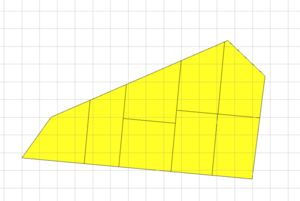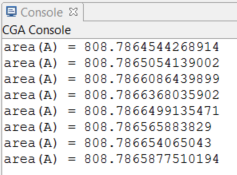In comparison, when using the split operation, the lots are different colors because the block is divided into lots with different areas. ``````Lot2 --> Split(3) Split(n) --> case n == 0 : B case scope.sz > scope.sx : split(z) { ~1 : Split(n-1) | ~1 : Split(n-1) } else : split(x) { ~1 : Split(n-1) | ~1 : Split(n-1) } B --> color(getColor) print("area(B) = " + geometry.area)``````